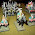## Monday, 29 April 2013

### Probability for Wargamers 11 - combinations and Chain of Command

Oi bin finkin', like....

The Lardies' Chain of Command has an interesting activation mechanic, and I've been pondering for a while what the odds are on various things happening as a result. To start with, we need to figure out the  probability of rolling a given number a certain number of times on 5d6. So let's start with the easy case.

What's the probability of rolling NO 1s on 5d6?

Easy!

Chance of not rolling a 1 = 5/6.
Chance of rolling 5 'not 1's' = 5/6 * 5/6 * 5/6 * 5/6 * 5/6 = 3125/7776 = roughly 40%!

How about probability of rolling one 1 on 5d6?

Chance of rolling a 1 = 1/6
Chance of not rolling a 1 = 5/6
Chance of rolling a 1 then 4 x 'not 1' = 1/6 * 5/6 * 5/6 * 5/6 * 5/6 = 625/7726 = about 8.1%.

Except that there are actually 5 different ways we can do this: the first, second... etc etc dice can be the 1. As these are independent events, we can add them, giving us a probability of roughly 40%.

Simple enough, that's 1/6 * 1/6 * 5/6 * 5/6 * 5/6 * the number of different ways we can do that...

Which is 125/7776 * ... erm...

Right...

So...

We have 5 ways we can place the first 1. And for each of those, we have 4 different places we can put the other 1. So that's 5x4 = 20...

...except that since we don't care about the order, a dice in position 1 followed by a dice in position 2 is exactly the same as the reverse, so in fact there are only 10. Which makes the odds of rolling exactly 2 ones 125/7776 * 10, or 16% or so.

It turns out this 'pick k from n items' thing has a name, and a formula, which is going to save our sanity working through this. What isn't going to save my sanity here is that neither of the conventional ways of writing it is easy on a blog: however, with a little HTML wizardry....

The number of combinations of n things taken k at a time,

nCk = (n * (n-1) ... * (n-k+1) ) / (k * (k-1) * (k-2) ...)

As you can see, for our example above, and for the rest...
5C2 = (5 x 4) / (2 x 1) = 10 - odds of 2 1's = 16.1%
5C3 = (5 x 4 x 3) / (3 x 2 x 1) = 10 - odds of 3 1's = 10 x 25 / 7776 = 3.2%
5C4 = (5 x 4 x 3 x 2) / (4 x 3 x 2 x 1) = 5 - odds of 4 1's = 5 x 5 / 7776 = 0.3%
and obviously
5C5 = 1 - odds of 5 1's = 1/7776 = 0.01%

I think that car's relatively safe, Rich :D

Now we've got that, we can apply some maths to various things in Chain of Command.

See you next time for that!

1.This is where we get into the binomial theorem:
(A+B)^n = nCn.A^n + nCn-1.A^(n-1).B + nC(n-2).A^(n-2).B^2 + ... + nCr.A^(r).B^(n-r) + ... + nC1.A.B^(n-1) + nCo.B^n

This is a very useful equation when determining multiples of either/or or hit/miss outcomes. (A+B)^n = 1, simply means that you will get a bunch of 'hits' and/or a bunch of 'misses'; no other result is possible. The expamsion might look like a waste of time, but they give you the number (n) of hits, say.

(1/6 + 5/6)^5 expands to
(1/6)^5 + 5.(1/6)^4.(5.6) + 10.(1/6)^3.(5/6)^2
+ 10.(1/6)^2.(5/6)^3 + 5.(1/6).(5/6)^4 + (5/6)^5.
Each of those terms (0-5) equals the probability of rolling 0-5 '1's (or any given number) on a D6.

5 '1's = 1/7776
4 '1's = 25/7776
3 '1's = 250/7776
2 '1's = 1250/7776
1 '1' = 3125/7776
0 '1's = 3125/7776

To check my maffs you simply add up the numerators, which should come out at 7776. They do. So you are more likely to roll at least one '1' than not; you are as likely to roll exactly 1 '1' as none at all; the probability of rolling more than 1 '1' is very nearly 1 in 5 (4 to 1 against).

It is sometimes useful to determine the 'expected score' which involves term by term multiplying each probability by the actual number of 'hits' it represents. In the above example, your 'expected score' (ES) is about 0.73. It has to be admitted that it's not a particularly useful finding for this particular example, but it can be in other circumstances.

For example, suppose you had several units - lets say it's 4 - firing, each receiving 5 dice to roll, '1's to 'hit'. Then as the ES is about 73%, we can reckon we can expect overall to score 73% x 4 'hits' - call it 3. We might score more, slightly more likely less (the miniscule chance of scoring 20 hits tends to skew the results). But we can feel unlucky if we score less than 2 hits, and very fortunate indeed if we roll more than 4.

Cheers,
Ion

2.Blimey.
Thanks Mike, I was having trouble getting to sleep so thought I'd catch up on your blog. Now my brain is melting.
Cheers anyway.

Views and opinions expressed here are those of the commenter, not mine. I reserve the right to delete comments if I consider them unacceptable. Unfortunately due to persistent spam from one source, I've been forced to turn on captchas for comments.

Comments on posts older than 7 days will go into a moderation queue.